Homework Help Question & Answers

# Design an analogue clock using Matlab. Designed using Matlab Instructions: 1. The clock you are designing...

Design an analogue clock using Matlab.
Designed using Matlab

Instructions:
1. The clock you are designing should have the same function as the real-life clock.

2. It has hour hand, minutes hand, second hand with different length.

3. The hour hand, minutes hand, second hand rotates clockwise direction.
4. It should have numbers 1-12 on the clock.

5. Hour hand fully rotate clockwise from 12 to 12 for 12 hour. Minute hand fully rotate clockwise from 12 to 12 for 60 minute. Second hand fully rotate clockwise from 12 to 12 for 60 seconds.

6. Include steps and thinking process in the scricpt using comment (%%).

7. Display date if possible. (Bonus Point)

#### Homework Answers

Answer #1

`Hey,

Note: Brother if you have any queries related the answer please do comment. I would be very happy to resolve all your queries.

clc;clear;clf;
format shortg;
x=0;y=0;r=10;
hold on;
th = 0:pi/50:2*pi;
xunit = r * cos(th) + x;
yunit = r * sin(th) + y;
h = plot(xunit,yunit);
r=8; s=;
for th = pi/6: pi/6: 2*pi
ytemp = r * cos(th);
xtemp = r * sin(th);
text(xtemp,ytemp,num2str(s));
s = s+1;
end
while(1)
c = clock;
c = c(1,4:6);
min =c(1,2); sec=c(1,3);
if (c(1,1)>12)
hr = c(1,1)-12;
else
hr = c(1,1);
end
min1 = ceil(min/12);
theta = (hr*pi)/6 + (min1*pi)/30;
f=figure(1); hold on;
ytemp = 3 * cos(theta); Y = [0 ytemp];
xtemp = 3 * sin(theta); X = [0 xtemp];
p=plot(X,Y);hold on;
theta1 = (min*pi)/30;
ytemp1 = 5 * cos(theta1); Y1 = [0 ytemp1];
xtemp1 = 5 * sin(theta1); X1 = [0 xtemp1];
p1=plot(X1,Y1);
theta2 = (sec*pi)/30;
ytemp2 = 7 * cos(theta2); Y2 = [0 ytemp2];
xtemp2 = 7 * sin(theta2); X2 = [0 xtemp2];
p2=plot(X2,Y2);
pause(1);
delete(p2);
delete(p1);
delete(p);
end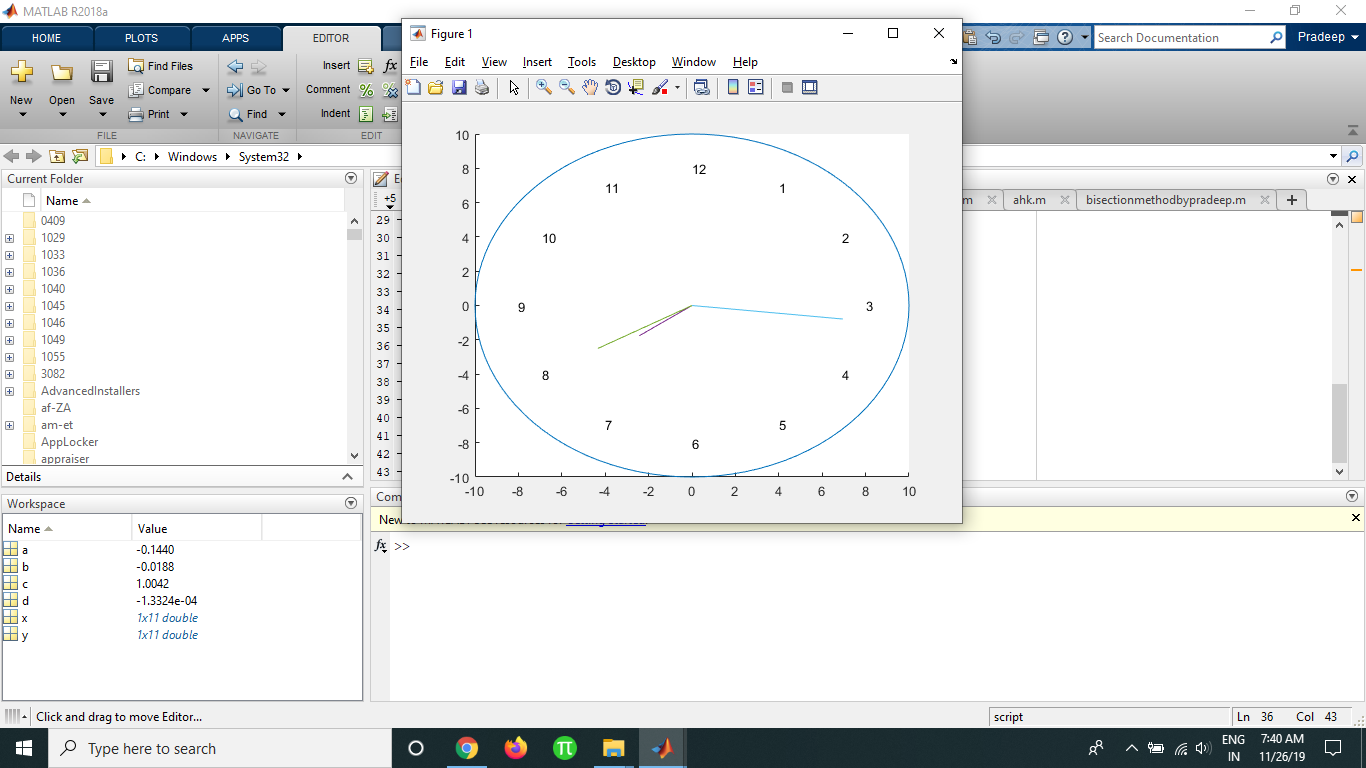Kindly revert for any queries

Thanks.

Know the answer?
Your Answer:

#### Post as a guest

Your Name:

What's your source?

#### Earn Coin

Coins can be redeemed for fabulous gifts.

Not the answer you're looking for? Ask your own homework help question. Our experts will answer your question WITHIN MINUTES for Free.
Similar Homework Help Questions
• ### Design a way of using the pushbutton to trigger an interrupt to your system. Your system,...

Design a way of using the pushbutton to trigger an interrupt to your system. Your system, upon receiving this interrupt, responds by reading and displaying the temperature in Fahrenheit degrees on the LCD display in the format of xxx.x. The system must resume displaying the digital clock. Build your circuit and include all the required information about your hardware design in your lab report. 4. Based on the hardware you designed, choose an interrupt/timer tool from mbed to update the...

• ### MATLAB code help! Function Name: chemTimer Inputs: 1. (double) The current position of the hour hand 2. (double) The current position of the minute hand 3. (double) A positive or negative number of m...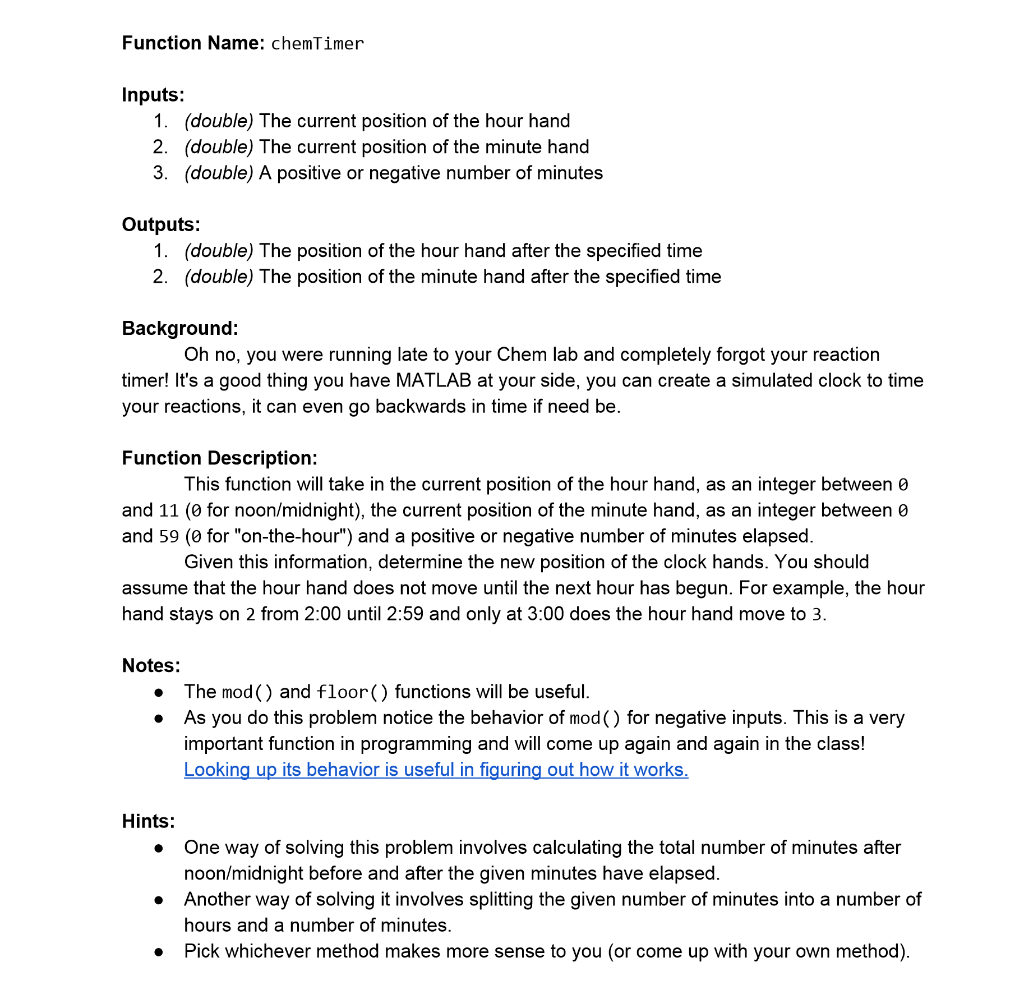MATLAB code help! Function Name: chemTimer Inputs: 1. (double) The current position of the hour hand 2. (double) The current position of the minute hand 3. (double) A positive or negative number of minutes Outputs: 1. (double) The position of the hour hand after the specified time 2. (double) The position of the minute hand after the specified time Background: Oh no, you were running late to your Chem lab and completely forgot your reaction timer! It's a good thing...

• ### Hey there, I am having a hard time using GUI for c# in visual studio. I...

Hey there, I am having a hard time using GUI for c# in visual studio. I am making an alarm clock. I have a few questions that I would like to know how to do: --using a button called set time to capture text from a box containing a user-inputted hour, minute, second, and setting the current time to that. --using a button called alarm, Capture the Text in the TextBoxes - the Hours, Minutes, Seconds and Text (am/pm) and...

• ### MATLAB Qla In the fib_clock.m file, write a function file that accepts the hour and minute values, and returns the logical vectors that indicate the appropriate Fibonacci numbers (1, 1, 2, 3, 5]...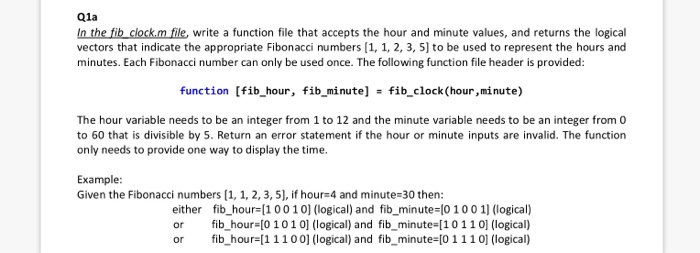MATLAB Qla In the fib_clock.m file, write a function file that accepts the hour and minute values, and returns the logical vectors that indicate the appropriate Fibonacci numbers (1, 1, 2, 3, 5] to be used to represent the hours and minutes. Each Fibonacci number can only be used once. The following function file header is provided: function [fib_hour, fib minute] fib_clock(hour,minute) The hour variable needs to be an integer from 1 to 12 and the minute variable needs to...

• ### HI C PROGRAMMING COULD YOU,, Rewrite the program to have it display the time on the...

HI C PROGRAMMING COULD YOU,, Rewrite the program to have it display the time on the second line of the display, using the HH:MM:SS format. Use the 24-hour format for the hours, in other words, have the time go from 00:00:00 to 23:59:59. #include<LiquidCrystal.h> LiquidCrystal LcdDriver(11, 9, 5, 6, 7, 8); int minutes = 27; //These global integers keep the value of the clock int sec = 10; int hr = 10; const long interval = 1000; //This interval is...

• ### Problem 1 [35 points] A large clock on the wall has an hour hand, a minutes...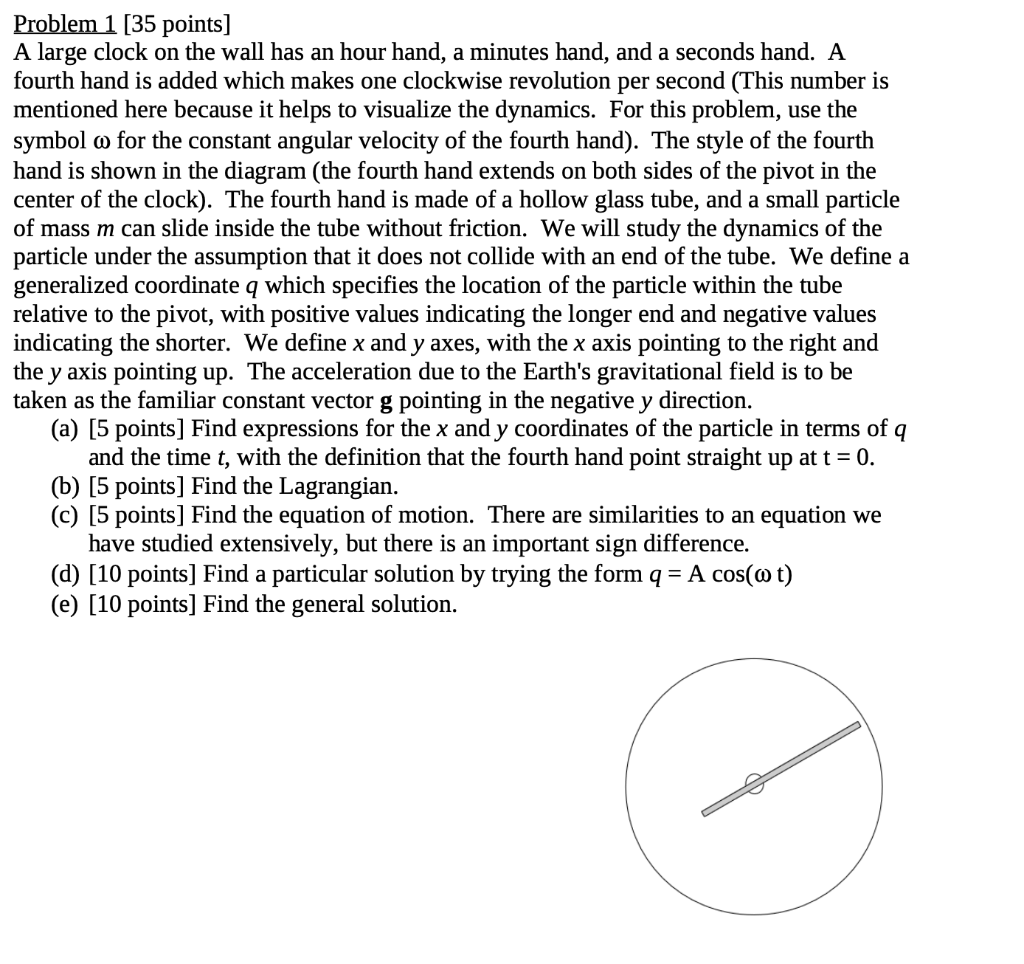Problem 1 [35 points] A large clock on the wall has an hour hand, a minutes hand, and a seconds hand. A fourth hand is added which makes one clockwise revolution per second (This number is mentioned here because it helps to visualize the dynamics. For this problem, use the symbol o for the constant angular velocity of the fourth hand). The style of the fourth hand is shown in the diagram (the fourth hand extends on both sides of...

• ### Lab Exercise #11 Assignment Overview You will work with a partner on this exercise during your la...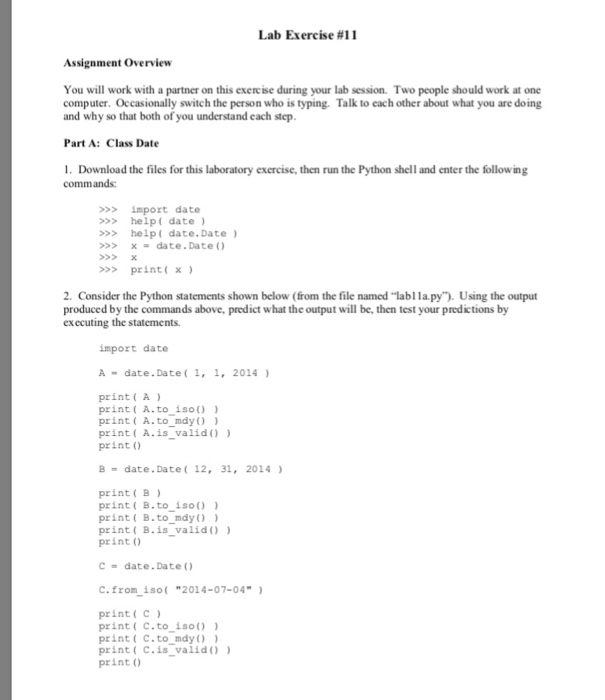help with cse problem Lab Exercise #11 Assignment Overview You will work with a partner on this exercise during your lab session. Two people should work at one computer. Occasionally switch the person who is typing. Talk to each other about what you are doing and why so that both of you understand each step Part A: Class Date . Download the files for this laboratory exercise, then run the Python shell and enter the following commands: >>>import date >>help(...

• ### You want to design a digital watch using the private timer. Write the code with ARM assembly and C. Make your own decision on the language selection (Assembly and C). The followings are the codin...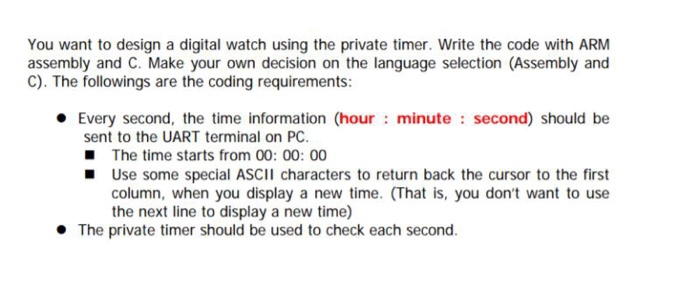You want to design a digital watch using the private timer. Write the code with ARM assembly and C. Make your own decision on the language selection (Assembly and C). The followings are the coding requirements: Every second, the time information (hour minute second) should be sent to the UART terminal on PC The time starts from 00: 00: 00 Use some special ASCII characters to return back the cursor to the first column, when you display a new time....

• ### Write in C++ please In Chapter 10, the class clockType was designed to implement the time...

Write in C++ please In Chapter 10, the class clockType was designed to implement the time of day in a program. Certain applications, in addition to hours, minutes, and seconds, might require you to store the time zone. Derive the class extClockType from the class clockTypeby adding a member variable to store the time zone. Add the necessary member functions and constructors to make the class functional. Also, write the definitions of the member functions and the constructors. Finally, write...

• ### MATLAB Questions. I have a basic understanding of the tools for MATLAB, but do not know...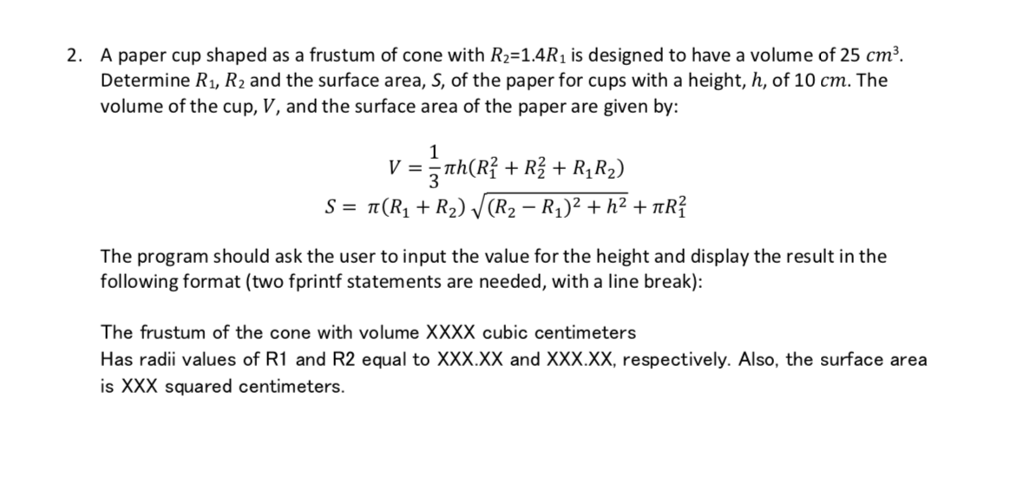MATLAB Questions. I have a basic understanding of the tools for MATLAB, but do not know how to create the code for these questions. Please show the entire code. 2. A paper cup shaped as a frustum of cone with R-14R1 is designed to have a volume of 25 cm Determine R1, R2 and the surface area, S, of the paper for cups with a height, h, of 1O cm. The volume of the cup, V, and the surface area...